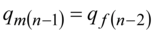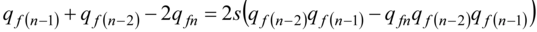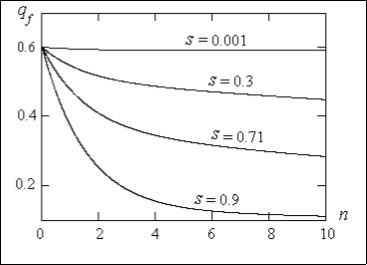### Journal of Genetic Engineering

Current Issue Volume No: 1 Issue No: 1

ISSN: 2694-1198

Mini Review Open Access
• Available online freely Peer Reviewed
• ### Natural Selection in a Population is a Problem of Nonlinear Genetics

A.N. Volobuev 1

1Samara State Medical University, Department of Medical Physics, Samara, Russia

## Abstract

The problem of natural selection against recessive homozygotes in a population is investigated. It is shown that natural selection of mutant alleles linked with the Х-chromosome in a population at women is described by the nonlinear differential equation of the third order. The order of the differential equation characterizes a power of selection. It is marked that the high order of the differential equation of natural selection allows level all mutational processes in Earth populations.

##### Author Contributions
Received 02 Nov 2019; Accepted 01 Dec 2019; Published 04 Dec 2019;

Academic Editor: Angela Pia Cazzolla, professor for the Master degree of Dentistry and for the in Pediat Vocational Masters Degree ric Dentistry and Dental Traumatology at University of Foggia , Italy.

Checked for plagiarism: Yes

Review by: Single-blindThis is an open-access article distributed under the terms of the Creative Commons Attribution License, which permits unrestricted use, distribution, and reproduction in any medium, provided the original author and source are credited.

Competing interests

The authors have declared that no competing interests exist.

Citation:

A.N. Volobuev (2019) Natural Selection in a Population is a Problem of Nonlinear Genetics. Journal of Genetic Engineering - 1(1):10-14.

## Introduction

In 1 it is shown that variation of the recessive (or dominant) genes linked to theХ-chromosome frequency during time of a population life in condition of balance of a population is described by the linear differential equation of the second order. This equation expresses Hardy – Weinberg law for panmictic population. Similar processes can be attributed to processes of linear genetics.

However in genetics there are the processes resulting in the nonlinear differential equations. These processes concern to nonlinear genetics.

The problem of mutant allele natural selection has been mentioned for a family tree in 2. The basic characteristics of selection have been introduced: parameter of selection s and fitness of a genotype 1 - s .

Fitness of a genotype characterizes ability (probability) of the given genotype to a reproduction.

We accept the ratio of fitnesses of genotypes АА, Аа, аа2:

1:1:(1 - s). ……..(1)

In the formula (1) s there is standard parameter of selection against of the recessive homozygotes.

In 2 the simplified technique of algebraic interrelation reception between the recessive allele frequency q f at women and dimensionless time n at presence of selection has been used.

In the given article we shall solve a problem of selection in a population using a standard way of transition from finite-differential equation characteristic for a family tree to the differential equation correct for a population 1.

As well as in 2 the analysis of natural selection we shall carry out by the example of selection against of the recessive homozygotes.

In 2 has been received the recurrent ratio for the finding of a recessive allele frequency in the following women generation for autosomes:

……...(2)

Genotypes linked to theХ-chromosome at women after selection we shall write down for the generation n - 1 2:

……….(3)

Taking into account that

……..(4)

we find the sum of the genotypes frequencies in formula (3): 1 - qm(n-1)qf(n-1)s.

Further, using a standard rule of an alleles frequency finding in the following generation (half of heterozygotes frequency plus of homozygotes frequency) and the formula (3) we calculate the frequency of recessive alleles a at women in generation n for sex-linked genes:

………..(5)

Let's transform the formula (5) using pm(n-1) = 1 - qm(n-1) and pf(n-1) = 1 - qf(n-1). For recessive allele frequency at women in Х-chromosomes the formula (5) is transformed:

……..(6)

Besides it is necessary to take into account the major condition that allele frequency in the Х-chromosome of the women is equal to frequency of same alleles at the men qm in the following generation. For recessive allelea this condition looks like:……..(7)

The condition (7) is caused theХ-chromosome of the woman at inheritance (mating) passes to the male offspring.

Substituting (7) in (6) we find:

……..(8)

By simple algebraic transformations of the formula (8) we shall find:…….(9)

Let’s consider the differential equation:……..(10)

where η and α are some constants. Differentiation in (10) goes on dimensionless time n (normalized on average time of generation alternation in a population).

Let’s pass in (10) to finite-differential form of derivatives:……..(11)

Transforming (11) by analogy to 1 we shall find:……...(12)

Let’s identify the equation (12) with the equation (9). In 1 as a result of a similar identification the size η = 3/2Δn has been found. Besides it is possible to see that α = - 1/2Δn2.

Hence, the differential equation (10) can be written down as:

........(13)

At transition to a population the number of generation of a role does not play therefore, we shall receive:……..(14)

The nonlinear differential equation (14) allows calculate frequency qf of intact recessive allele at women in the Х-chromosomes of a population at presence of selection on mutant alleles.

For convenience of calculation it is used in (14) new independent variable t = n/Δn √s/2. If to accept Δn = 3/2Ln 21, we shall find t = 2 LN 2n/3 √s/2 ≈ 0.462n √s/2 .

In view of the new independent variable the equation (14) will be transformed to the kind:

……...(15)

where it is designated Ԑ=3/√2s. At variation of a selection standard parameter 0≤s≤1 the size. ∞≥Ԑ≥3/√2 ≈ 2.12

The nonlinear differential equation (15) can be solved only numerically.

Initial conditions, first of all, are necessary for the solution of the differential equation (15): initial frequency qf0 of the recessive allele a, and initial speed of falling of this frequency (dqf/dt)0 or (dqf/dn)0.

Initial frequency qf0 at t=0 or n=0 was accepted qf0=0.6. Initial speed of falling of the allele frequency was accept (dqf/dt)0 = -0.6 or (dqf/dn)0 =-0.165. The step of calculation on a variable t equal Δt=0.001, and numbers of steps 20000 was used.

On Figure 1 the graphs of dependence of intact recessive allele frequency qf in Х-chromosomes at women in a population on dimensionless time n are shown at various parameters of selection s.

Figure 1. The dependence of intact recessive allele frequency in Х-chromosomes at women in a population on dimensionless time at various parameters of selectionIn conclusion we shall consider the some general-biological questions connected to functioning of natural selection.

There is very high degree of nonlinearity - the third - of the differential equation (15) describing natural selection in populations. It has important general-biological importance. The degree of nonlinearity of natural selection defines its opportunities, i.e.power of selection. We did not was success to find out the equations of mutational processes with higher degree of nonlinearity. The inbred mutational processes have the second degree of nonlinearity 3.

Other mutational processes, for example, resulting to hemophilia or induced mutagenesis under action of radiation have the first degree of nonlinearity or in other words these processes are linear. It means that any mutational processes in populations of the Earth can be levelled by natural selection. From this point of view the hypothesis of H. Muller 4 about danger of the future biological degeneration of mankind owing to accumulation of a genetic load for the account of inbreeding is hardly a correct hypothesis.

Damage of a genome by mutational processes in earthly populations can cause destruction of a considerable part of individuals, including people. It is the extremely unpleasant and with it is necessary to resist in the various ways: creation of new medical products, protection from ionizing radiations, etc. But as a whole these negative processes practically do not threaten populations, since natural selection gradually restores genetic norm in a population.

But it is possible to assume a fantastic situation that to the Earth there arrive some technologically high-civilized individuals from other planet able to operate genic mechanisms. These individuals can start mutational process in a human population of fourth or higher order of nonlinearity. Now it is difficult to assume concrete character of such process. However, completely clearly that the natural selection generated in the earthly conditions cannot reset similar mutational influence. Earthly populations will begin to die out completely and the faster the higher the degree of nonlinearity of the given hypothetical mutational process. And to intervene in this process the populations with lower technological capabilities of a manipulating the genofund is not capable.